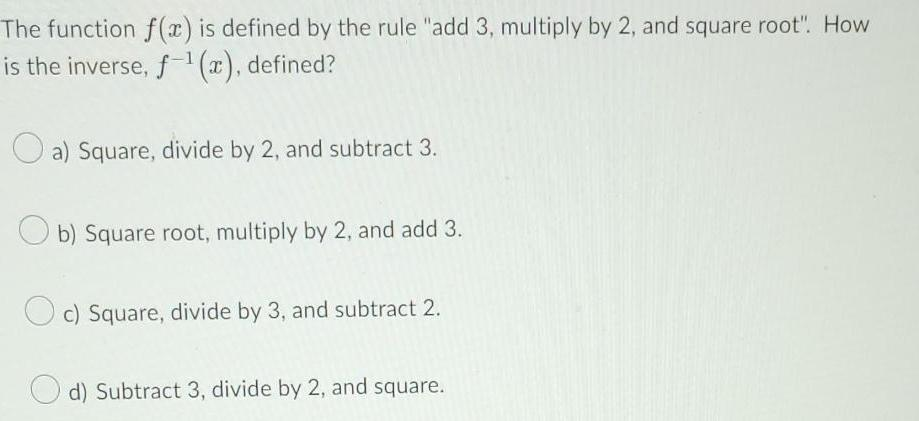Question:

# The function f(x) is defined by the rule "add 3, multiply by

Last updated: 8/11/2022The function f(x) is defined by the rule "add 3, multiply by 2, and square root". How is the inverse, f¹(x), defined? a) Square, divide by 2, and subtract 3. Ob) Square root, multiply by 2, and add 3. c) Square, divide by 3, and subtract 2. d) Subtract 3, divide by 2, and square.